# AP Board 6th Class Maths Solutions Chapter 1 Numbers All Around us InText Questions

AP State Syllabus AP Board 6th Class Maths Solutions Chapter 1 Numbers All Around us InText Questions and Answers.

## AP State Syllabus 6th Class Maths Solutions 1st Lesson Numbers All Around us InText QuestionsWrite the numbers in expanded form. (Page No. 5)

Question 1.
96,08,54,039
Solution:
96,08,54,039 = 9 × 10,00,00,000 + 6 × 1,00,00,000 + 8 × 10,00,000 + 5 × 10,000 + 4 × 1000 + 3 × 10 + 9 × 1
Ninety six crores eight lakhs fifty four thousand and thirty nine.

Question 2.
857,90,00,756
Solution:
857,90,00,756 = 8 × 100,00,00,000 + 5 × 10,00,00,000 + 7 × 1,00,00,000 + 9 × 10,00,000 + 7 × 100 + 5 × 10 + 6 × 1
Eight hundred fifty seven crores ninety lakhs seven hundred and fifty six.

1 Crore = 10 Ten Lakhs
= 100 Lakhs
= 1000 Ten Thousands
= 10,000 Thousands
= 1,00,000 Hundreds
= 10,00,000 Tens
= 1,00,00,000 Unit’s

Check Your Progress (Page No. 6)

Question 1.
Write 10 crores and 100 crores as in the above table.
Solution:
Ten crores = 10 One crores
= 100 Ten lakhs
= 1000 Lakhs
= ,10,000 Ten thousands
= 1,00,000 Thousands
= 10,00,000 Hundreds
= 1,00,00,000 Tens
= 10,00,00,000 UnitsHundred crores = 100 One crores
= 10 Ten crores
= 10,000 Lakhs
= 1.0. 000 Ten thousands
= 10.0. 000 Thousands
= 1.0. 00.000 Hundreds
= 10.0. 00.000 Tens
= 100.0. 00.000 Units

Check Your Progress (Page No. 8)

Question 1.
Write remaining numbers of the above table in the International System.
Solution: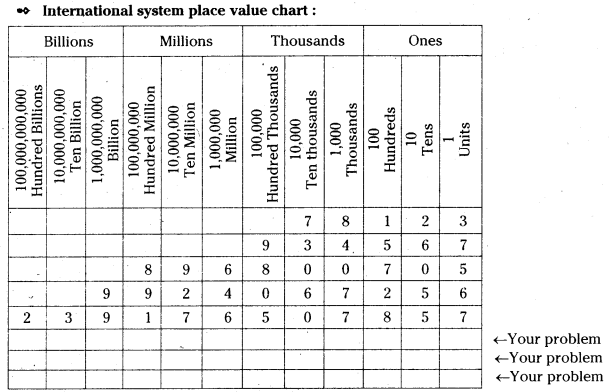Question 2.
Fill the boxes in the table with your own numbers and write in words in the International system.
Solution:
a) 896800705

Put comma for each period 896,800,705 in International System.
In expanded form :
= 8 ×x 1,000,000,000 + 9 × 10,000,000 + 6 × 1,000,000 + 8 × 100,000 + 7 × 100 + 5 × 1

In word form :
Eight hundred ninety six millions eight hundred thousand seven hundred and five.b) 239176507857
Put comma for each period 239,176,507,857 in International System.
In expanded form :
= 2 × 100,000,000,000 + 3 × 10,000,000,000 + 9 × 1,000,000,000 + 1 × 100,000,000 + 7 × 10,000,000 + 6 × 1,000,000 + 5 × 100,000 + 7 × 1,000 + 8 × 100 + 5 × 10 + 7 × 1
In word form :
Two hundred thirty nine billion one seventy six million five hundred seven thousand eight hundred and fifty seven.

c) 452069258932
Put comma for each period 452,069,258,932
In expanded form :
= 4 × 100,000,000,000 + 5 × 10,000,000,000 + 2 × 1,000,000,000 + 6 × 10,000,000 + 9 × 1,000,000 + 2 × 100,000 + 5 × 10,000 + 8 × 1,000 + 9 × 100 + 3 × 10 + 2 × 1
In word form :
Four hundred fifty two billion sixty nine million two hundred fifty eight thousand nine hundred and thirty two.

d) 839241367054
Put comma for each period 839,241,367,054
In expanded form :
8 × 100,000,000,000 + 3 × 10,000,000,000 + 9 × 1,000,000,000 + 2 × 100,000,000 + 4 × 10,000,000 + 1 × 1,000,000 + 3 × 100,000 + 6 × 10,000 + 7 × 1.000 + 5 × 10 + 4 × 1
In word form :
Eight hundred thirty nine billion two hundred forty one million three hundred sixty seven thousand and fifty four.

e) 342056743298
Put comma for each period 342,056,743,298
In expanded form :
3 × 100,000,000,000 + 4 × 10,000,000,000 + 2 × 1,000,000,000 + 5 × 10,000,000 + 6 × 1,000,000 + 7 × 100,000 + 4 × 10,000 + 3 × 1,000 + 2 × 100 + 9 × 10 + 8 × 1
In word form :
Three hundred forty two billion fifty six million seven hundred forty three thousand two hundred and ninety eight.

Question 1.
Round off each to the nearest ten, hundred and thousands.
(1) 56,789 (2) 86,289 (3) 4,56,726 (4) 5,62,724
Solution:

 S.No. Number Nearest ten Nearest hundred | Nearest thousand 1. 56,789 56,790 56,800 57,000 2. 86,289 86,290 86,300 86,000 3. 4,56,726 4,56,730 4,56,700         ’ 4,57,000 4. 5,62,724 5,62,720 5,62,700 5,63,000

Let’s Explore (Page No.12)

Question 1.
Discuss with your friends about rounding off numbers. Consider the population of A.P., Telangana and India in 2011. Round off the numbers to the nearest lakhs.
Solution:

 State Population in 2011 Round off the nearest lakhs Andhra Pradesh 4,92,94,020 4,93,00,000 Telangana 3,52,86,757 3,53,00,000 India 1,21,08,54,977 1,21,09,00,000Estimate the sum by rounding and verify the result. (Page No.12)

Question 1. 8756 + 723
Solution:
Given 8756 + 723
First estimate by rounding = 8800 + 700 = 9500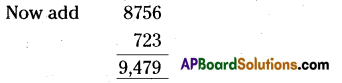Thus sum is 9,479.
Think
9479 is close to the estimate of 9500.

Question 2.
56723 + 4567 + 72 + 5
Solution:
Given 56723 + 4567 + 72 + 5
First estimate by rounding = 56720 + 4570 + 70 + 10 = 61370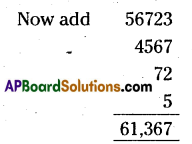The sum is 61,367.
Think
61367 is close to the estimate of 61370.

Question 3.
656724 + 8567
Solution:
Given 656724 + 8567
First estimate by rounding = 657000 + 9000 = 666000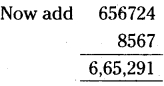The sum is 6,65,291.

Think
665291 is close to the estimate of 666000.

Question 4.
60756 + 2562 + 72
Solution:
Given 60756 + 2562 + 72
First estimate by rounding = 60760 + 2560 + 70 = 63390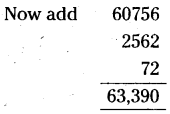The sum is 63,390.
Think
63390 is equal to the estimate of 63390.Estimate the difference by rounding and verify the result.Pg. No. 13)

Question 1.
7023 – 856
Solution:
Given, 7023 – 856
First estimate by rounding = 7000 – 900 = 6100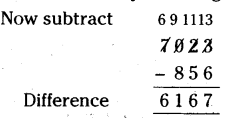Think
6167 is close to the estimate of 6100

Question 2.
9563 – 2847
Solution:
Given, 9563 – 2847
First estimate by rounding = 10000 – 3000 = 7000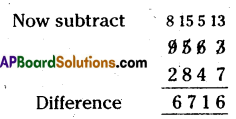Think
6716 is close to the estimate of 7000

Question 3.
52007 – 6756
Solution:
Given, 52007 – 6756
First estimate by rounding = 52000 – 7000 = 45000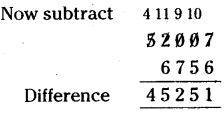Think
45251 is close to the estimate of 45000Question 4.
95625 – 4235
Solution:
Given, 95625 – 4235
First estimate by rounding = 95600 – 4200 = 91400 .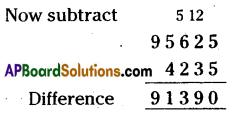Think
91390 is close to the estimate of 91400.

Estimate the product by rounding and verify the result.

Question 1.
63 × 85
Solution:
Given, 63 × 85
First estimate by rounding = 60 × 90 = 5400,
Rounding the result to hundreds = 5400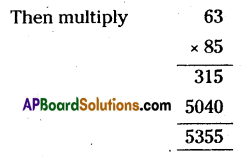Think
5355 is close to the estimate of 5400.

Question 2.
636 × 78
Solution:
Given, 636 × 78
First estimate by rounding = 640 × 80 = 51200
Rounding the result to hundreds = 51200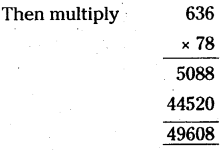Think
49608 is close to the estimate of 51200.

Question 3.
506 × 85
Solution:
Given, 506 × 85
First estimate by rounding = 500 × 90 = 45000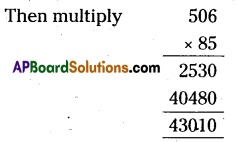Think
43010 is close to the estimate of 45000.

Question 4.
709 × 98
Solution:
Given, 709 × 98
First estimate by rounding = 700 × 100 = 70000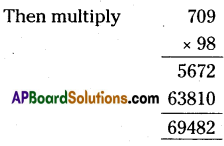Think
69482 is close to the estimate of 70000.Estimate the quotient by rounding and verify the result.

Question 1.
936 ÷ 7
Solution:
Given, 936 ÷ 7
Divide 936 ÷ 7
First estimate by rounding 1000 ÷ 10 = 100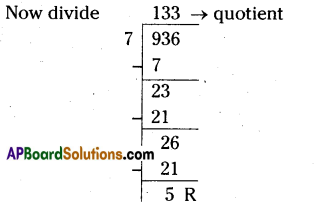Think
133 is close to the estimate of 100.

Question 2.
956 ÷ 17
Solution:
Given, 956 ÷ 17
Divide 956 ÷ 17
First estimate by rounding 1000 – 20 = 50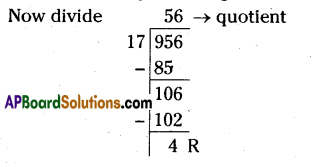Think
56 is close to the estimate of 50.

Question 3.
859 ÷ 23
Given, 859 ÷ 23
Divide 859 ÷ 23
First estimate by rounding 860 ÷ 20 = 43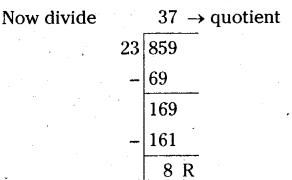Think
37 is close to the estimate of 43.Question 4.
708 ÷ 32
Given, 708 ÷ 32
Divide 708 ÷ 32
First estimate by rounding 710 ÷ 30 = 23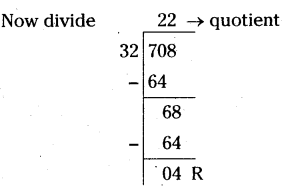Think
22 is close to the estimate of 23.